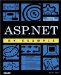# CompareValidator Control

 I l @ ve RuBoard

### CompareValidator Control

Often the validity of an item on a form can only be determined based on its relationship to other values on the form. For example, a form might ask the users how much money they would like to donate to a charity, and then ask them how much of their donation they would like to put toward, say, .NET programming training (it could happen ). It wouldn't make any sense for the second value to exceed the first value because it is limited by the total amount the user has donated. In situations like these, the CompareValidator can be used to ensure that the relationships between controls on a form are valid. Table 8.5 describes the properties that make the CompareValidator different from the BaseValidator class discussed earlier.

##### Table 8.5. CompareValidator Class Additional Properties
Method Type Description
ControlToCompare String The control to which the ControlToValidate should be compared.
Operator ValidationCompareOperator The type of comparison to be performed. One of: DataTypeCheck , Equal, GreaterThan , GreaterThanEqual , LessThan , LessThanEqual , NotEqual .
Type ValidationDataType The data type of value in the ControlToValidate . Must be one of the following: Currency , Date , Double , Integer , String .
ValueToCompare String Can be used instead of ControlToCompare to set a comparison value at design time.

As with the RangeValidator , we must specify a Type . This is because the comparison only makes sense if the two values are of the same data type. The kind of comparison must also be chosen , as the Operator property, and should be fairly self-explanatory. The DataTypeCheck is the only one that bears explanation. This value is simply used to ensure that the data type of the ControlToValidate is the same as that of the ControlToCompare (or ValueToCompare ). Let's look at a simple example of how to use the CompareValidator before going into any more detail. Listing 8.3 demonstrates how to force a user to enter a total amount to donate, followed by a smaller or equal amount to put toward .NET programmer training. The validator will simply display a "*" if the values are incorrect. Figure 8.3 shows the output.

##### Figure 8.3. Example0803.aspx with invalid values.##### Listing 8.3 : Adding compare validation to a text box.` <%@ Page %> <HTML>     <HEAD>         <!DOCTYPE HTML PUBLIC "-//W3C//DTD HTML 4.0 Transitional//EN" >     </HEAD>     <body>         <form method="post" runat="server">             <asp:Label ID="errorMessage" Runat="server"></asp:Label>             <table>                 <tr>                     <td>                         Total Donation:                     </td>                     <td>                         <asp:textbox runat="Server" columns="6"                                                  id="total_donation">  </asp:textbox>                     </td>                     <td>                         <asp:requiredfieldvalidator runat="Server"     id="total_donation_required" controltovalidate="total_donation"     display="dynamic" errormessage="A total donation value is required.">                             *                         </asp:requiredfieldvalidator>                         <asp:rangevalidator runat="Server"     id="total_donation_range" controltovalidate="total_donation"     display="dynamic" errormessage="Total dontation must be between  <%@ Page %> <HTML> <HEAD> <!DOCTYPE HTML PUBLIC "-//W3C//DTD HTML 4.0 Transitional//EN" > </HEAD> <body> <form method="post" runat="server"> <asp:Label ID="errorMessage" Runat="server"></asp:Label> <table> <tr> <td> Total Donation: </td> <td> <asp:textbox runat="Server" columns="6" id="total_donation"> </asp:textbox> </td> <td> <asp:requiredfieldvalidator runat="Server" id="total_donation_required" controltovalidate="total_donation" display="dynamic" errormessage="A total donation value is required."> * </asp:requiredfieldvalidator> <asp:rangevalidator runat="Server" id="total_donation_range" controltovalidate="total_donation" display="dynamic" errormessage="Total dontation must be between \$0 and \$5000." minimumvalue="0" maximumvalue="5000" Type="Currency"> * </asp:rangevalidator> </td> </tr> <tr> <td> Portion of above donation to apply toward .NET programmer training: </td> <td> <asp:textbox runat="Server" columns ="6" id="net_portion"></asp:textbox> </td> <td> <asp:requiredfieldvalidator runat="Server" id="net_portion_required" controltovalidate="net_portion" display="dynamic" errormessage="A .NET portion value is required."> * </asp:requiredfieldvalidator> <asp:CompareValidator Runat="server" ID="net_portion_compare" ControlToValidate="net_portion" ControlToCompare="total_donation" Operator="LessThanEqual" ErrorMessage="You cannot apply more toward .NET training than your total donation." Display="Dynamic" Type="Currency"> * </asp:CompareValidator> <asp:CompareValidator Runat="server" ID="net_portion_positive" ControlToValidate="net_portion" ValueToCompare="0" Type="Currency" Display="Dynamic" Operator="GreaterThanEqual" ErrorMessage=".NET portion must be a positive value."> * </asp:CompareValidator> </td> </tr> </table> <asp:button id="save_button" runat ="Server" text="Save"></asp:button> </form> </body> </HTML>  and 00."     minimumvalue="0" maximumvalue="5000" Type="Currency">                         *                         </asp:rangevalidator>                     </td>                 </tr>                 <tr>                     <td>                         Portion of above donation to     apply toward .NET programmer training:                     </td>                     <td>                         <asp:textbox runat="Server" columns="6"     id="net_portion"></asp:textbox>                     </td>                     <td>                         <asp:requiredfieldvalidator runat="Server"     id="net_portion_required" controltovalidate="net_portion" display="dynamic"     errormessage="A .NET portion value is required.">                             *                         </asp:requiredfieldvalidator>                         <asp:CompareValidator Runat="server"     ID="net_portion_compare" ControlToValidate="net_portion"     ControlToCompare="total_donation" Operator="LessThanEqual"     ErrorMessage="You cannot apply more toward .NET training than your total donation."     Display="Dynamic" Type="Currency">                         *                         </asp:CompareValidator>                         <asp:CompareValidator Runat="server"     ID="net_portion_positive" ControlToValidate="net_portion" ValueToCompare="0"     Type="Currency" Display="Dynamic" Operator="GreaterThanEqual"     ErrorMessage=".NET portion must be a positive value.">                         *                         </asp:CompareValidator>                     </td>                 </tr>             </table>             <asp:button id="save_button" runat="Server"     text="Save"></asp:button>         </form>     </body> </HTML> `

For completeness, we continue to use the Required and Range validators in this example. However, the important validation to notice is the CompareValidator , which enforces the rule that states that the net_portion control's value cannot exceed the total_donation control's value. This would not prevent net_portion from being negative, however. For this reason, we used the second CompareValidator , net_portion_positive , to ensure that the net_portion control's value was greater than or equal to 0. This is an example of using the CompareValidator without ControlToCompare .

In many situations, more complicated patterns will be involved. For example, you might think that the RangeValidator would work for validating ZIP codes or telephone numbers , but in reality these kinds of values are much better validated by using regular expressions. We will briefly examine regular expressions and the .NET RegularExpressionValidator control in the next section.

 I l @ ve RuBoardASP.NET by Example
ISBN: 0789725622
EAN: 2147483647
Year: 2001
Pages: 154

Similar book on Amazon